Comment
Search
Duplicate
Try Notion
3.2 Efficiency Among RAL Estimators
Now that we’ve gotten rid of all the estimators we don’t want (i.e. those that aren’t RAL), we need to figure out how to sort through the all remaining estimators we might have at our disposal:
So let's do just that. By imposing asymptotic linearity, we've actually made our job super easy. As $n$﻿ gets bigger and bigger, we know that the sampling distribution of any RAL estimator $\hat\psi_k$﻿ minus truth (scaled by root $n$﻿) goes to a normal with mean zero and variance given by $V[\phi_k(Z)]$﻿ where $\phi_k$﻿ is the influence function for that particular estimator. That means that the only difference between any two RAL estimators (in large enough samples) is that they have sampling variances of different magnitude. Obviously we would prefer an estimator with less variance because that will give us smaller (but still valid) confidence intervals and p-values. In other words, given the same data, we are more certain of our estimate if we use an estimator that has a smaller sampling variance.
The implication is that, if given the choice, we want to pick an estimator with an influence function that has the smallest possible variance.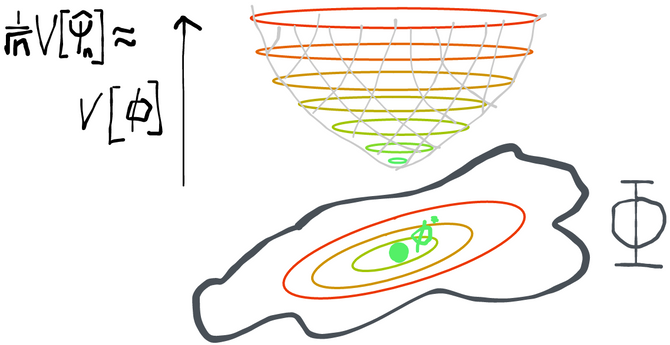However, instead of picking an estimator out of a lineup, why don't we make our own estimator that is guaranteed to beat anything that anyone else could come up with? Here is a strategy that will let us do that:
Figure out the set of all possible influence functions: $\Phi = \{\phi: \phi \text{ is an IF for some RAL estimator}\}$﻿
Pick the one that has the smallest variance: $\phi^\dagger = \argmin_{\phi \in \Phi} V[\phi]$﻿
Build an estimator that has that influence function.
This section will cover steps 1 and 2 of this strategy. There are a few different strategies to build an estimator that has the influence function with smallest variance and these will be the subject of the next chapter.
It's important to come away with an understanding of what and the why of what we're going to discuss. In practice, unless you're working with brand-new model spaces or parameters, you will never have to actually do any of what is described in this section. Nonetheless it's critical to understand it to have an idea of how the tools we have today were developed and why they work.
Characterizing the Set of Influence Functions
Not every possible function corresponds to a valid RAL estimator in a particular statistical model. For example, it would be great if we could find a RAL estimator with $\phi(Z) = 0$﻿ because this estimator would have no variance at all in large samples. Clearly that's a pipe dream that's not going to happen in general, so we must conclude that $\phi = 0$﻿ is not an influence function. For a given parameter and statistical model, what functions $\phi(Z)$﻿ are influence functions of RAL estimators, and what functions aren't?
Using nothing but the definitions of regularity and asymptotic linearity, we arrive at the following result. For any RAL estimator with influence function $\phi$﻿, the following holds for all scores $h$﻿ corresponding to paths in the model:
$\lim_{\epsilon \rightarrow 0} \frac{\psi(\tilde P_\epsilon) - \psi(P)}{\epsilon} = E[\phi h]$
Before giving a proof, which sadly relies on some technical results, let's understand what this is even saying. The term on the left is what we call the pathwise derivative of $\psi$﻿ at $P$﻿ in the direction $h$﻿ (evaluated at 0). From now on we'll abbreviate this with the notation $\nabla_h \psi(P)$﻿. The term on the right is the covariance between $h(Z)$﻿ and $\phi(Z)$﻿ because both are mean-zero. So what the result above says is that
🌈
[Reisz representation, central identity for influence functions] If $\phi$﻿ is an influence function for a RAL estimator of a parameter $\psi$﻿ and $h$﻿ is the score for any legal path at $P\in \mathcal M$﻿, the $h$﻿-direction pathwise derivative of $\psi$﻿ is equal to the covariance of $h$﻿ and $\phi$﻿: $\nabla_h \psi = E[\phi h]$﻿
This is a fascinating connection between what seem to be two very different things. The pathwise derivative describes how quickly the estimand (parameter) changes as we move along a particular path. The expectation of the influence function times the score effectively tells us what the angle is between the path's score and the estimator's influence function (see section below on the space $\mathcal L_2$﻿ if this confuses you). The left-hand side (pathwise derivative) depends on the parameter $\psi$﻿, the direction $h$﻿, and the true distribution $P$﻿. The right hand side (influence function covariance w/ score) depends on the choice of estimator, implying $\phi$﻿, the direction $h$﻿, and the true distribution $P$﻿ (under which the covariance is taken).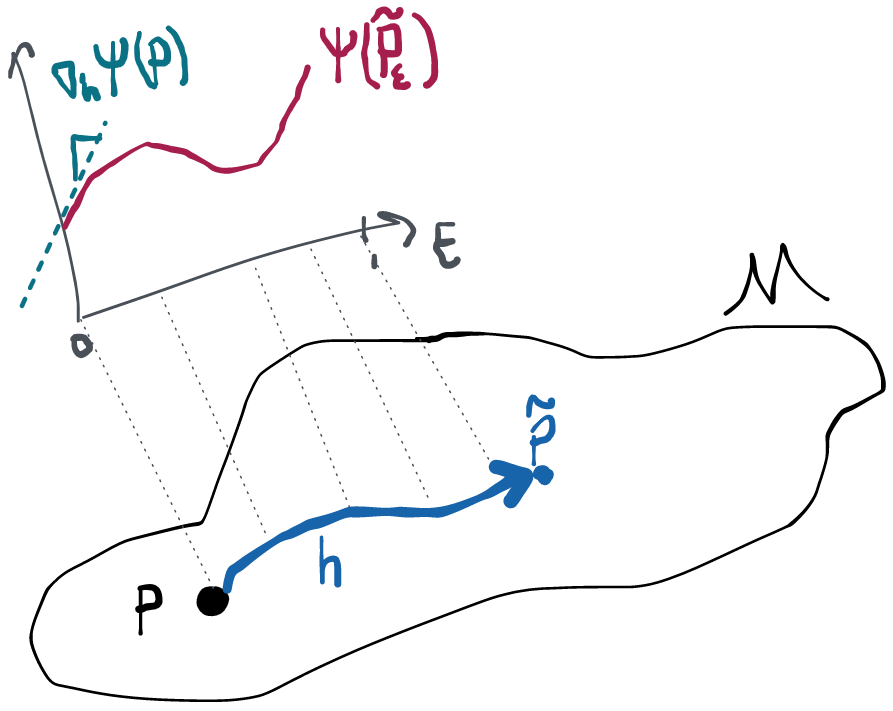This is cool in and of itself, but it's also the fundamental key to characterizing the set of all influence functions, and therefore the key link that holds everything we're talking about together.
😱 Proof that $\nabla_h \psi = E[h\phi]$﻿
We'll start with our definition of a path and our definition of asymptotic normality. Using just these definitions and some esoteric theorems, we'll amazingly be able to characterize how our estimator should behave as we move along the path towards $P$﻿. That's a little surprising because asymptotic normality is a property that only holds at $P$﻿ and doesn't say anything explicit about behavior along paths. Nonetheless, we'll see that there is an implication for how the estimator behaves along paths. However, we've also assumed the estimator is also regular, which is already a definition of how the estimator behaves along paths. In comparing the derived behavior from asymptotic normality and the assumed behavior from regularity, we will see there is a difference. The difference in the two behaviors can only be made to go away (as it must if an estimator is both asymptotically normal and regular) if $\nabla_h \psi = E[h\phi]$﻿, so that's what we conclude.
In proving that asymptotic normality and our definition of a path by themselves imply some behavior of the estimator along such a path we'll have to use two advanced, technical results. Unfortunately I haven't found an alternative way to prove this that is both rigorous and intuitive. It's not at all impossible to understand these theorems (just read the appropriate sections of vdW 1998) but if you're not interested in the math for its own sake it's probably not worth your time. In terms of understanding the arc of the proof you just need to grasp how we've used these tools to get what we need, not how they work.
Throughout I'll abbreviate $\hat\psi_n =\hat\psi(\mathbb P_n)$﻿ (the estimate as we draw increasing samples from $P$﻿) and $\psi = \psi(P)$﻿ (the true parameter at $P$﻿) to keep the notation light. I'll also abbreviate $\hat{\tilde\psi}_n =\hat\psi(\tilde{\mathbb P}_{1/\sqrt n})$﻿, which is the estimate as we draw increasing samples from distributions moving along our path closer to $P$﻿, and $\tilde\psi_n = \psi(\tilde P_{1/\sqrt n})$﻿, which are the true parameter values at each of these distributions. Anything that has a hat is an estimate, anything that has a tilde is along a path.
Alright, go time. Let's see what we can say about how our estimator behaves along sequences of distributions like $\tilde P_n = \tilde P_{\epsilon = 1/\sqrt{n}}$﻿, since these are the only ones regularity has anything to say about. By theorem 7.2 of vdV 1998 the fact that our paths are differentiable in quadratic mean ensures that we get something called local asymptotic normality 🤷:
$\log \frac{ d\tilde P_{1/\sqrt n}^n }{ dP^n }(Z_1, \dots Z_n) = \frac{1}{\sqrt n}\sum^n h(Z_i) - \frac{1}{2}E[h(Z)^2] + o_P(1)$
It really doesn't matter what this means because we're just going to use it to satisfy a particular technical condition in a minute. The important part is to see that we've defined some random variable on the left: the derivative-looking thing is just some fixed function of the data, so let's rename that as some random variable $T_n$﻿. What the right hand says is that in large-enough samples, this $T_n$﻿ thing looks like a sum of IID variables plus some constant. We combine this with the assumed asymptotic linearity of our estimator:
$\sqrt{n}(\hat\psi(\mathbb P_n) - \psi(P)) = \frac{1}{\sqrt n}\sum^n\phi(Z_i) + o_P(1)$
Which is also a random variable that's approximately equal to an IID sum when $n$﻿ gets big. Here we stack the two above equations on top of each other and by the central limit theorem we have that
$\left[ \begin{array}{c} T_n \\ \sqrt n (\hat\psi_n -\psi) \end{array} \right] \overset{P}\rightsquigarrow \mathcal N \left( \left[ \begin{array}{c} -\frac{1}{2}P[h^2] \\ 0 \end{array} \right] , \left[ \begin{array}{cc} P[h^2] & P[h\phi] \\ P[h\phi] & P[\phi^2] \\ \end{array} \right] \right)$
Now we use another technical result 🧐 (Le Cam's 3rd lemma- see vdV 1998 ex. 6.7), which says that when we have exactly the situation above, we can infer
\begin{align*} \sqrt n \left(\hat{\tilde \psi}_n - \psi \right) &\overset{\tilde P_{1/\sqrt n}}{\rightsquigarrow} \mathcal N\left(P[h \phi], P[\phi^2]\right) \end{align*}
Again it's not important to understand the technical device. The idea is that we're trying to say something about how our estimator behaves as we change the distribution along our path of interest. At first this seems impossible because asymptotic normality only holds at each $P$﻿ and doesn't say anything about what happens along paths. However, with the help of these technical devices, we've actually managed to say something about the difference between the estimate as we change the underlying distribution and the truth at $P$﻿. In particular, this difference converges to a normal with the same variance as if we had not been moving along the path towards $P$﻿ but had instead sat still at $P$﻿. Crazy. The limiting normal distribution now has some mean $P[h\phi]$﻿, which we'll pull out in the course of some algebraic manipulation during which we also add and subtract $\sqrt n \tilde\psi_n$﻿ on the left:
\begin{align*} \sqrt n \left(\hat{\tilde \psi}_n - \psi \right) &\overset{\tilde P_{1/\sqrt n}}{\rightsquigarrow} \mathcal N\left(P[h \phi], P[\phi^2]\right) \\ -P[h \phi] + \sqrt n \left( \hat{\tilde \psi}_n - \tilde\psi_n + \tilde\psi_n - \psi \right) &\overset{\tilde P_{1/\sqrt n}}{\rightsquigarrow} \mathcal N \left( 0, P[\phi^2] \right) \\ \end{align*}
Moving terms around, we arrive at
$\left[ \sqrt n \left( \tilde\psi_n - \psi \right) - P[h \phi] \right] + \sqrt n \left( \hat{\tilde \psi}_n - \tilde\psi_n \right) \overset{\tilde P_{1/\sqrt n}}{\rightsquigarrow} \mathcal N \left( 0, P[\phi^2] \right) \tag{AL}$
Notice that the term in brackets on the left is a constant for each $n$﻿, i.e. it's not random.
Now, finally, we recall our definition of regularity, which was
$\sqrt n \left( \hat{\tilde \psi}_n - \tilde\psi_n \right) \overset{\tilde P_{1/\sqrt n}}{\rightsquigarrow} \mathcal N \left( 0, P[\phi^2] \right) \tag{R}$
An estimator that is asymptotically linear must satisfy the behavior in the display above denoted (AL). An estimator that is regular must satisfy the behavior in the display denoted (R). A RAL estimator must satisfy both. But the only way that both of those can be true is if they are actually saying the same thing, which only happens if the bracketed term in (AL) goes to zero. Thus, for RAL estimators,
\begin{align*} \lim_{n\rightarrow \infty}\sqrt n \left( \tilde\psi_n - \psi \right) &= P[h \phi] \\ \lim_{\epsilon_n \rightarrow 0} \frac{ \tilde\psi_n - \psi }{ \epsilon_n } &= P[h \phi] \\ \nabla_h \psi &= P[h \phi] \end{align*}
where we've recalled $\epsilon_n = 1/\sqrt{n}$﻿ from our original definition of our sequence along the path.
What we mean is this: since the left-hand side doesn't depend on the influence function $\phi$﻿, the same identity holds (keeping constant $h$﻿ and $P$﻿) for any two estimators with influence functions $\phi_1$﻿, $\phi_2$﻿ (if two such estimators exist). Specifically: $E[\phi_1 h] = \nabla_h \psi = E[\phi_2 h]$﻿. Eliminating the middleman, we get $E[(\phi_1 - \phi_2)h] = 0$﻿. Therefore the difference of influence functions for any two RAL estimators is orthogonal to any score! If you don't understand why this is the implication, you should review the section on $\mathcal L_2$﻿ space. Moreover, if we take any function $h^\perp$﻿ that is orthogonal to all scores and add it to the influence function from a RAL estimator, the result $\phi + h^\perp$﻿ still satisfies the above requirement and so this is an influence function for a different RAL estimator.
The Space $\mathcal L_2(P)$﻿
We can treat any score $h$﻿ and any influence function $\phi$﻿ as an element of a set we call $\mathcal L_2(P)$﻿ (or just $\mathcal L_2$﻿ when the measure $P$﻿ is clear). This is the set of all functions $f(Z)$﻿ which satisfy the condition $\int f(Z)^2 dP < \infty$﻿ (i.e. $f(Z)$﻿ is a random variable with finite variance). This space is a lot like the vector space $\mathbb R^p$﻿ in many important ways:
$\mathcal L_2$﻿ is a linear space: $f,g \in \mathcal L_2, \alpha \in \mathbb R \implies f + \alpha g \in \mathcal L_2$﻿
$\mathcal L_2$﻿ has an inner product: in $\mathbb R^p$﻿, the inner product between two vectors $x$﻿ and $y$﻿ is given by $\sum x_i y_i$﻿. The equivalent in $\mathcal L_2$﻿ for two functions $f$﻿ and $g$﻿ is $\int fg dP = E[fg]$﻿. Note how the integral of a product of two functions is a lot like the sum of the element-wise product of vectors. It happens that the two operations obey all the same rules that define something as an "inner product". When we don't have a specific space in mind, we usually write the inner product between two elements as $\langle u, v \rangle$﻿. If two elements have an inner product equal to 0 we say that the two are orthogonal. This generalizes the notion of two vectors being at a right angle in $\mathbb R^p$﻿. More generally, the inner product also defines what the "angle" is between two vectors: $\theta_{u,v} = \cos^{-1}\left(\frac{\langle u, v \rangle}{||v|| ||u||}\right)$﻿, where $||u|| = \langle u,u \rangle$﻿ is the norm of $u$﻿ in this space.
$\mathcal L_2$﻿ is complete. This is a technical term that means that the space contains all of its limit points (i.e. a sequence of convergent elements in the space can't converge to a limit that is outside the space).
Together, these conditions are the definition of something called a Hilbert space. Indeed, $\mathbb R^p$﻿ and $\mathcal L_2$﻿ are the usual examples of Hilbert spaces. The main difference between the two is that $\mathbb R^p$﻿ is finite-dimensional, whereas $\mathcal L_2$﻿ is infinite-dimensional. What do I mean by this? Well, a vector in, say $\mathbb R^3$﻿ clearly has 3 components $[x_1, x_2, x_3]$﻿. A "vector" in $\mathcal L_2$﻿ has as many components as its functions have arguments because you can think of a function $f:\mathbb R \rightarrow \mathbb R$﻿ like this: $f = [\dots f(-200) \dots f(-0.1) \dots f(0) \dots f(1) \dots f(1.5) \dots ]$﻿. The only difference is that I put the "vector index" in parentheses instead of as a subscript and now I call it an "argument". We also now allow for indices that are anywhere in the domain of $f$﻿ instead of just the integers $1,2,3$﻿. Alternatively, we can think of the vector $x$﻿ as a function that maps $i \in \{1,2,3\} \mapsto x_j$﻿. So vectors, functions, whatever. It's kind of the same thing in most of the ways that matter!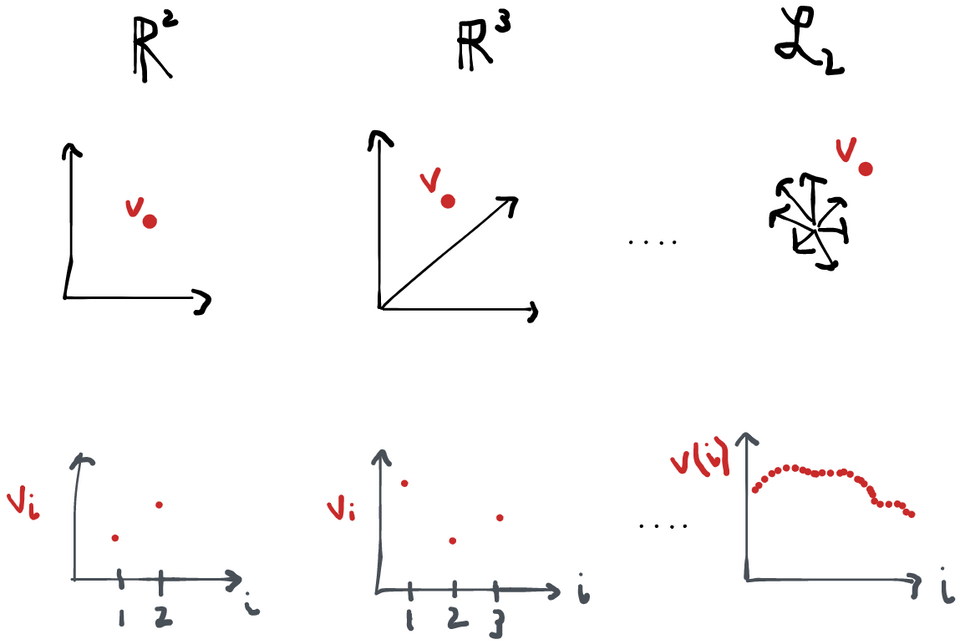Tangent Spaces
If influence functions are orthogonal to every score $h$﻿, they must also be orthogonal to any linear combination of scores, or any limit of a sequence thereof. So it makes everything more concise if we start talking about the tangent space $\mathcal T$﻿ (which is exactly the set of all scores, their linear combinations, and limits) instead of having to continually refer to "all scores". Since the tangent space comes from the set of scores, it has nothing to do with either the estimator or the parameter. It depends purely on the statistical model and the true distribution $P$﻿.
Why is it called the tangent space?
Honestly, I don't think it's the greatest name, but let's explain it.
The key is to 1) think about each point $P \in \mathcal M$﻿ as having density $p$﻿ w.r.t. some dominating measure $P\degree$﻿ and 2) realize that $p \in \mathcal L_2$﻿. Because $p$﻿ has to integrate to 1, then then norm of $\sqrt{p}$﻿ (that is, $E_{P\degree}[{\sqrt p \sqrt p}])$﻿ is of course 1. So we can identify $\mathcal M$﻿ with some subset of the unit ball in $\mathcal L_2(P\degree)$﻿. It's now easy to show that $\sqrt p$﻿ is orthogonal to $h \sqrt p$﻿:
$\int \sqrt p (\sqrt p h) dP\degree = \int h p dP\degree = \int hdP = 0$﻿
so the set of functions $h\sqrt p$﻿ ranging over scores $h$﻿ is orthogonal (tangent) to the point $\sqrt p$﻿ in the model space. Or, equivalently, $p$﻿ is orthogonal to all scores $h$﻿ in the space $\mathcal L(P\degree)$﻿.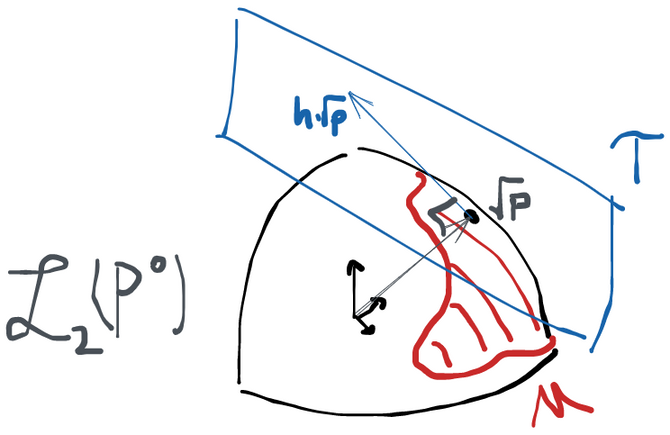Once nice thing that this picture shows is that the tangent space clearly depends on where $P$﻿ is within the model.
Nonetheless, it'd probably be more informative to call it the "score space", since the fact that the scores are tangent to the density $p$﻿ in $\mathcal L_2(P^\degree)$﻿ doesn't seem matter that much in terms of the role the space ends up playing in the theory we're developing. Alas, we're stuck with the names we have.
Saturated vs. Non-Saturated Models
Sometimes the tangent space is all of $\mathcal L_2^0$﻿. This happens if we put no restrictions on the distributions in our statistical model. If any distribution can be in the model, then starting at any point $P$﻿, any function $h$﻿ that has zero mean and finite variance defines a valid path at $P$﻿ because $\tilde p_\epsilon = (1+\epsilon h)p$﻿ will still be a density for any such score $h$﻿.
When this happens, we say that the model is nonparametric saturated, or just saturated (at $P$﻿). Intuitively, this means that we have a model space such that, standing at $P$﻿, we can move in any direction and still stay inside the model. If this is not the case, then we say the model is not saturated (at $P$﻿).
Factorizing Tangent Spaces
While we're on the subject of tangent spaces, it turns out that if we can factorize our distribution $P(Y, X) = P(Y|X)P(X)$﻿ then all scores $h$﻿ end up being the sum of scores for each factor, treating these as living in statistical models of their own, i.e. $P(Y|X) \in \mathcal M_{Y|X}$﻿. Moreover, the scores for each factor end up being orthogonal, i.e. $h = h_X(x) + h_{Y|X}(x, y)$﻿ and $h_X \perp h_{Y|X}$﻿. Lastly, these scores satisfy $E[h_X] = 0$﻿ and $E[h_{Y|X}|X]=0$﻿. A short proof of all of is given below. This argument also generalizes to densities that have more than two factors (just factor one of the two factors).
Proof
Recall that $h = \frac{d}{d\epsilon} \log \tilde p_\epsilon \big|_{\epsilon=0}$﻿. For $\epsilon$﻿ small enough $\tilde p_\epsilon$﻿ is still in the model, so we can factor it into $\tilde p_{Z_2|Z_1} \tilde p_{Z_1}$﻿(omitting the $\epsilon$﻿ subscripts). The log of a product is the sum of logs and the derivative is linear over a sum, so we get $h(Z_1, Z_2) = \underbrace{ \frac{d}{d\epsilon} \log \tilde p_{Z_2|Z_1} \big|_{\epsilon=0} }_{h_2(Z_1, Z_2)} + \underbrace{ \frac{d}{d\epsilon} \log \tilde p_{Z_1} \big|_{\epsilon=0} }_{h_1(Z_1)}$﻿
Now we'd like to show that $h_2 \perp h_1$﻿ in $\mathcal L_2(P)$﻿.
For that we have to notice that $h_2$﻿ is a score at $p_{Z_2|Z_1}$﻿ in the nonparametric model $\mathcal M_{Z_2|Z_1} = \{\text{all densities of Z_2 given Z_1\}}$﻿ (by definition). If we start at $p_{Z_2|Z_1}$﻿ and move along a path defined by $h_2$﻿, the only way we stay within $\mathcal M_{Z_2|Z_1}$﻿is if $E[h_2|Z_1] =0$﻿. Otherwise the resulting perturbation will not be a density. Similarly, we need for $E[h_1] = 0$﻿.
Now $E[h_1 h_2] = E[E[h_1 h_2|Z_1]] = E[h_1(Z_1) E[h_2|Z_1]] = E[h_1 \cdot 0] = 0$﻿ and we've shown $h_1$﻿ and $h_2$﻿ are orthogonal.
We can also go the other way- if I propose $h_1$﻿ and $h_2$﻿ that satisfy the above, then $h = h_1 + h_2$﻿ must be a valid score at $P$﻿ in the original model.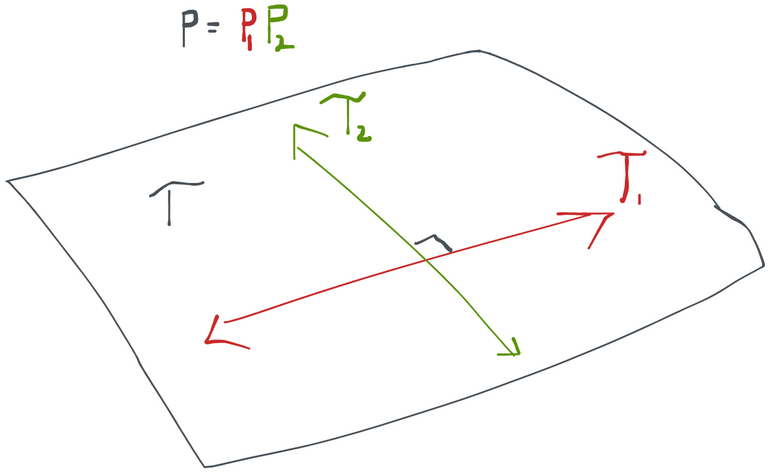Variational Independence
We showed that the tangent space can be broken up into an orthogonal sum when every distribution in the model factors and those factors can vary independently in their own model spaces. There are cases where this breaks down, though. If you look at the following picture, you'll surmise that all the scores at $P$﻿ (blue arrows) can indeed be created as orthogonal sums of scores from $\mathcal M_{Z_2|Z_1}$﻿ and $\mathcal M_{Z_1}$﻿. However, there are arrows that can be constructed the same way (grey, dotted) that take us outside of the model space- these are not scores because they don't correspond to a legal path inside the model. Therefore in this case $\mathcal T \subset \mathcal T_1 \oplus \mathcal T_2$﻿, but we don't have the equality. When this happens, we say that our submodels are not variationally independent. In other words, there are some points in the model space where I can't legally change in one of the submodels without having to make a change in another (i.e. at $P$﻿ in the picture we can't go just up, we also have to move a bit to the right along the diagonal).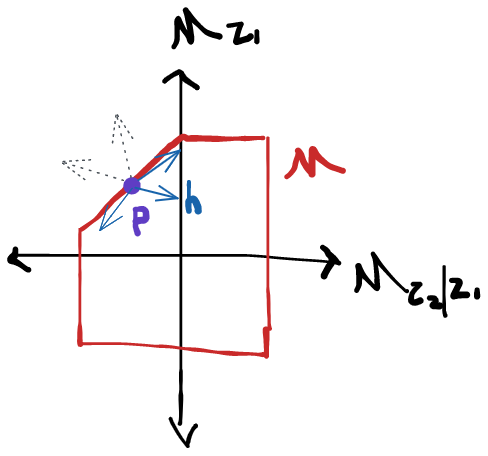We can therefore usually construct tangent spaces for each factor separately and then the tangent space for the whole model is the orthogonal sum of the component tangent spaces (we write this $\mathcal T = \mathcal T_1 \oplus \mathcal T_2$﻿).
Consider what happens if we perturb a density $p$﻿ along a path defined by a score $h_X$﻿ that satisfies $h_X(y,x) = h_X(x)$﻿ and $E[h_X] = 0$﻿. We know that $\tilde p_\epsilon(y,x) = (1+\epsilon h_X)p(y,x)$﻿, but what can we say about the resulting factors $\tilde p_\epsilon(y|x)$﻿ and $\tilde p_\epsilon(x)$﻿? A bit of algebra using the properties of $h_X$﻿ and the definition of the conditional density shows that $\tilde p_\epsilon(y|x) = p(y|x)$﻿ and also $\tilde p_\epsilon(x) = (1+\epsilon h_X)p(x)$﻿. In other words, moving along a path in $\mathcal M_X$﻿ only affects the factor $p(x)$﻿. Similarly, if you repeat this exercise with a score $h_{Y|X}$﻿ satisfying $E[h_{Y|X}|X] = 0$﻿ you get that $\tilde p_\epsilon(y|x) = (1+\epsilon h_{Y|X})p(y|x)$﻿ and also $\tilde p_\epsilon(x) = p(x)$﻿. Thus moving along a path in $\mathcal M_{Y|X}$﻿ only affects the factor $p(y|x)$﻿. This should make some intuitive sense to you, and, naturally, everything generalizes cleanly when there are more than two factors.
This is useful when we evaluate the directional derivative $\nabla_h \psi$﻿. If $h$﻿ is the sum of two other functions we can always write $\nabla_h\psi = \nabla_{h_X} \psi + \nabla_{h_{Y|X}}\psi$﻿ because the derivative is a linear operator in the score. The resulting terms $\nabla_{h_X} \psi$﻿ and $\nabla_{h_{Y|X}}\psi$﻿ are now easier to evaluate because for each of them we just need to consider a single perturbation in $p(x)$﻿ or in $p(y|x)$﻿, respectively. We’ll see this come in handy in the next section of this chapter.
Example: tangent space for a randomized controlled trial
Let's give a concrete example of a tangent space at $P$﻿ for some particular statistical model. For our model, we'll use the space $\mathcal M_{\text{RCT(\pi)}}$﻿ where RCT($\pi$﻿) stands for "randomized controlled trial" with treatment mechanism $\pi$﻿. This model is characterized by a joint distribution between some vector of observed covariates $X$﻿, a binary treatment $A$﻿, and an outcome $Y$﻿. Any distribution of three variables can always factor as $P(Y,A,X) = P(Y|A,X)P(A|X)P(X)$﻿. The treatment mechanism is defined as $\pi(X) = P(A=1|X)$﻿, the probability of receiving treatment given observed covariates $X$﻿.
What makes the RCT model space different from the more general nonparametric space where each of these three distributions can be anything is that in the RCT the distribution of $A|X$﻿ is fixed. Specifically, we know $P(A=1|X) = \pi(X)$﻿. For example, in a trial with a simple 50:50 randomization we have $\pi(X) = 0.5$﻿.
So what are the possible scores? The density factors into three components, only two of which can actually vary. Thus any score will be the sum of two orthogonal components, one of which corresponds to $p_{X} \in \mathcal M_X$﻿ and one of which corresponds to $p_{Y|A,X} \in \mathcal M_{Y|A,X}$﻿ (recall $p_{A|X}$﻿ is fixed so we can't move in that "direction").
Now we're basically done. Since there are no more restrictions on our model, our tangent space is orthogonal sum of the two tangent subspaces. Let $\mathcal L_2^0$﻿ be the subspace of all zero-mean functions in $\mathcal L_2$﻿ (recall all scores and all influence functions have mean zero). Now, formally:
\begin{align*} \mathcal T_X &= \left\{ h : \begin{split} &E[h]=0 \\ &h(Y,A,X) = h(X) \end{split} \right\} \\ \mathcal T_{Y|A,X} &= \left\{ h : E[h|A,X]=0 \right\} \\ \mathcal T_{\text{RCT}} &= \mathcal T_X \oplus \mathcal T_{Y|A,X} \end{align*}Can you think of a function that is in $\mathcal L_2^0$﻿ but not in $\mathcal T_{\text{RCT}}$﻿?
Example: tangent space for an observational study
The difference between the observational study model and the RCT model is that now we don't know the treatment mechanism $P(A|X)$﻿. This model (we'll call it $\mathcal M_\text{obs}$﻿) therefore contains $\mathcal M_{\text{RCT(\pi)}}$﻿ for any treatment mechanism $\pi$﻿.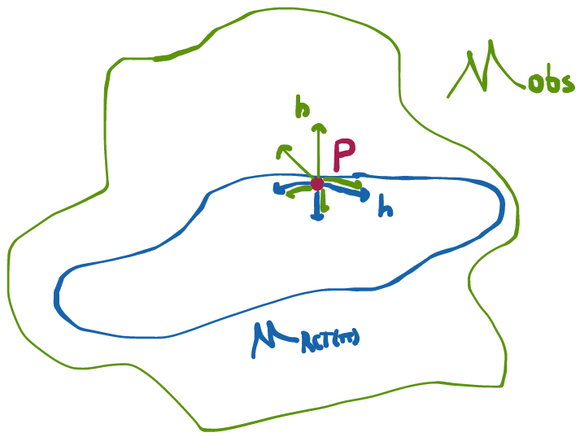Since this model is larger than $\mathcal M_{RCT}$﻿, it should make some intuitive sense to you that the tangent space is bigger too. This is because at any point $P$﻿ in the model, we have more directions we can move in than we previously did. Specifically, we can now move in directions $h$﻿ that end up changing the treatment mechanism. To be specific, we can have paths through $\mathcal M_{\text{obs}}$﻿ (specified by some $h$﻿) such that $\tilde P_\epsilon(A|X) \ne P(A|X) = \pi(X)$﻿. But this same $h$﻿ cannot be a path through $\mathcal M_{\text{RCT}(\pi)}$﻿ because for the fluctuated distribution $\tilde P_\epsilon$﻿ to remain inside of $\mathcal M_{\text{RCT}(\pi)}$﻿ we have to require that $\tilde P_\epsilon(A|X) = \pi(X)$﻿. Therefore for any $P$﻿ with some treatment mechanism $\pi$﻿, all paths through $P$﻿ in $\mathcal M_{\text{RCT}(\pi)}$﻿ are also paths through $P$﻿ in $\mathcal M_\text{obs}$﻿ but not vice-versa.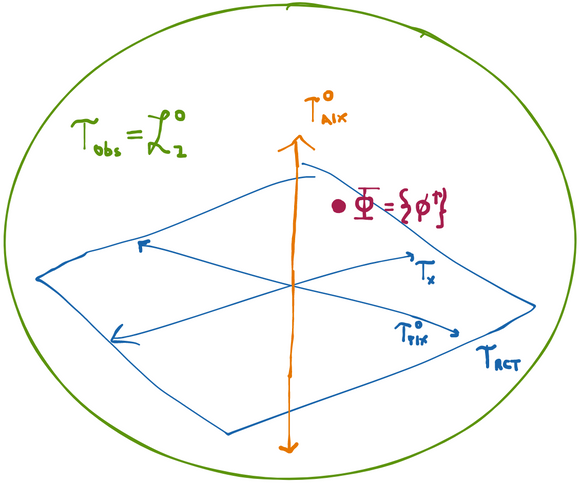We can be even more specific about this. Since any density in $\mathcal M_\text{obs}$﻿ factors as $P(Y,A,X) = P(Y|A,X)P(A|W)P(X)$﻿ we immediately have that the tangent space is given by the orthogonal sum $\mathcal T_{\text{obs}} = \mathcal T_{Y|X,A}^0 \oplus \mathcal T^0_{A|X} \oplus \mathcal T_{X}$﻿. Compare this to $\mathcal T_{\text{RCT}} = \mathcal T_{Y|X,A}^0 \oplus \mathcal T_{X}$﻿ and you immediately see that scores in the observational study have an additional set of degrees of freedom that the score in the RCT don't have. Namely: all directions in $\mathcal T^0_{A|X}$﻿. In fact, $T_{\text{obs}} = \mathcal L_2^0$﻿, which is to say that any mean-zero function with finite variance is a legal score for this model. That's because we've put literally no restrictions on what $P(Y,A,X)$﻿ can be so $\tilde p_\epsilon = (1+\epsilon h)p$﻿ is a legitimate density in our model for any such $h$﻿.
The Efficient Influence Function
Starting with nothing but the definition of a RAL estimator, we've shown that the set of influence functions of RAL estimators is (after shifting it to the origin) orthogonal to the tangent space. Since RAL estimators basically only differ by their variance (which is the variance of their influence functions), we get the best RAL estimator by finding one that has the influence function with the smallest variance. The point of everything we've done in the section above is that at least now we know the space we have to look in!
Thankfully, our characterization of the tangent space and the set of influence functions makes it easy to find the influence function with the smallest variance.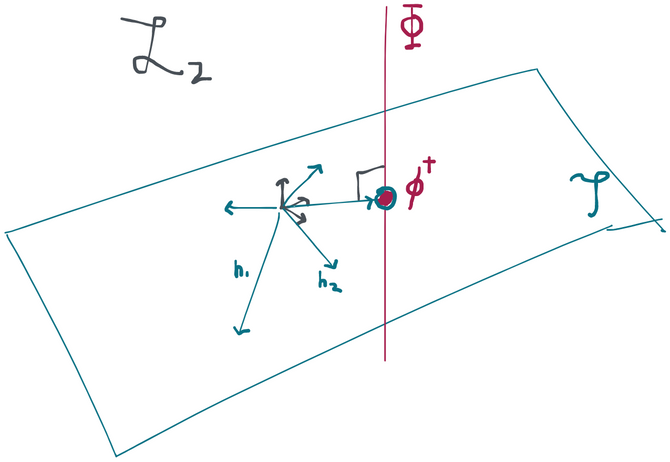Pathwise Differentiability
There are combinations of parameters and models for which the pathwise derivative doesn't exist or isn't a bounded linear operator. For example, consider densities of $Y,X$﻿ and let our parameter be $\psi(P) = E[Y |X=x_0]$﻿ for a particular point of interest $x_0$﻿. You can evaluate the derivative and show that $\nabla_h \psi(P) = \int y h(y,x_0)p_{Y|X}(y,x_0) dy$﻿. The problem here is that I can always pick $h$﻿ with norm 1 but that takes huge values at $x=x_0$﻿ (by compensating with large negative values at other $x$﻿). So I can pick $h$﻿ (in the unit ball) such that the integral blows up as large as I want. That's what "unbounded" means for a linear operator. The consequence of this is that the Reisz representation theorem no longer applies and we cannot guarantee that there is an element $\phi^\dagger$﻿ that is in the tangent space. This also destroys the argument based on the difference of two influence functions of RAL estimators because we can't guarantee that even a single RAL estimator exists.
To do this we can argue that there is some influence function that is itself in the tangent space because we can always project some influence function $\phi$﻿ into $\mathcal T$﻿ to get $\phi = \phi^\dagger + h^\perp$﻿. By definition, $\phi^\dagger$﻿ is in the tangent space, and by what we know about the relationship of $\Phi$﻿ to the tangent space, $\phi^\dagger$﻿ is the difference of an influence function with something that's orthogonal to all scores, meaning that it too must be an influence function. We can reach the same conclusion if we use the Reisz representation theorem. If the pathwise derivative, viewed as a function of the score $h$﻿, is bounded and linear among scores in $\mathcal T$﻿, then there is some element of $\mathcal T$﻿ that satisfies our requirement of being an influence function for a RAL estimator. This is is usually the case, although there are some parameters and models for which it isn't. But as long as we have pathwise differentiability, there is always exactly one influence function that is also in the tangent space.
Reisz Representation
Besides giving us a definition of orthogonality, Hilbert spaces are super nice to work in because of an important property called Reisz representability. The Reisz representation theorem says that if you have a bounded linear function $F$﻿ that maps an element of the Hilbert space to a real number, then that function can always be represented as an inner product between the argument to the function and some other element of the Hilbert space. In other words, for every bounded linear function $F$﻿, there is some element $h_F$﻿ so that $F(h) = \langle h_F, h \rangle$﻿.
This is a very surprising result at first but it's pretty easy to convince yourself of it in $\mathbb R^p$﻿. Consider vectors $\vec x, \vec y \in \mathbb R^p$﻿ (I'll use the vector arrow $\vec x$﻿ in this section to be very explicit). The definition of a linear function $F: \mathbb R^p \rightarrow \mathbb R$﻿ is that $F(\vec x + \alpha \vec y) = F(\vec x) + \alpha F(\vec y)$﻿ for any scalar $\alpha$﻿. Any linear function in this particular space is bounded so we don't need to worry about what that means. To show that $F(\vec x)$﻿ is actually just taking an inner product between $\vec x$﻿ and some other vector we can apply $F$﻿ to the unit vectors $\vec e_{1 \dots p}$﻿ and see what it returns. Say $F(\vec e_i) = a_i$﻿. Now we can express any vector as the weighted sum of the unit vectors $\vec x = \sum x_i\vec e_i$﻿, and by linearity of $F$﻿ notice now that $F(\vec x) = \sum x_iF(\vec e_i) = \sum x_i a_i = \langle \vec x, \vec a\rangle$﻿. The conclusion is that the operation of the function $F$﻿ on $\vec x$﻿ is really just the inner product between $\vec x$﻿ and some other vector $\vec a$﻿, which is also in $\mathbb R^p$﻿. The other direction is also obvious: given $\vec a$﻿, define $F_{\vec a}(\vec x) = \langle \vec a, \vec x\rangle$﻿ and since the inner product is a linear operation in both arguments, we have that the function is linear as desired.
What's amazing is that this property actually extends to any Hilbert space (i.e. a complete linear space with an inner product). In particular, if we have a bounded linear function $F$﻿ that maps functions $f \in \mathcal L_2$﻿ to real numbers, then we know there is some fixed function $g \in \mathcal L_2$﻿ such that $F(f) = E[fg]$﻿. If you're unfamiliar with functions that have functions as arguments you can think of $F$﻿ as "assigning" a number to each function $f$﻿.
Projection in Hilbert Space
Almost all Hilbert spaces contain other Hilbert spaces within them. For example, we can think of any plane in 3D space as a 2D space in its own right. If we add the restriction that the contained space must have the origin in it, we call it a subspace and a useful result follows. Namely: for every vector $v$﻿ in the Hilbert space $\mathcal H$﻿, there is always exactly one vector $v_*$﻿ in the desired subspace $\mathcal H_*$﻿ and a vector $v^\perp$﻿ that's orthogonal to every vector in $\mathcal H_*$﻿ such that $v = v_* + v^\perp$﻿. Since the projection is unique, it's easy to check if $v_*$﻿ is indeed a projection by simply checking (1) whether $v_* \in \mathcal H_*$﻿ and (2) whether $v - v_* \perp \mathcal H_*$﻿.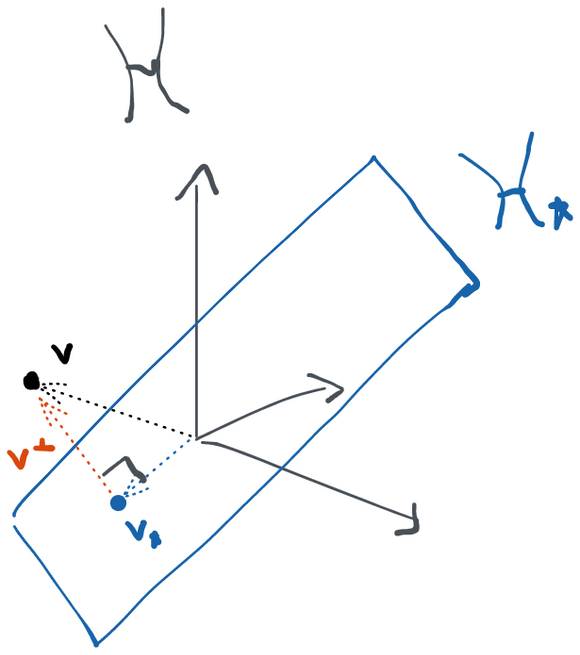If a subspace is the orthogonal sum of two other subspaces, we can always obtain the projection by projecting into each sub-subspace and then summing the result.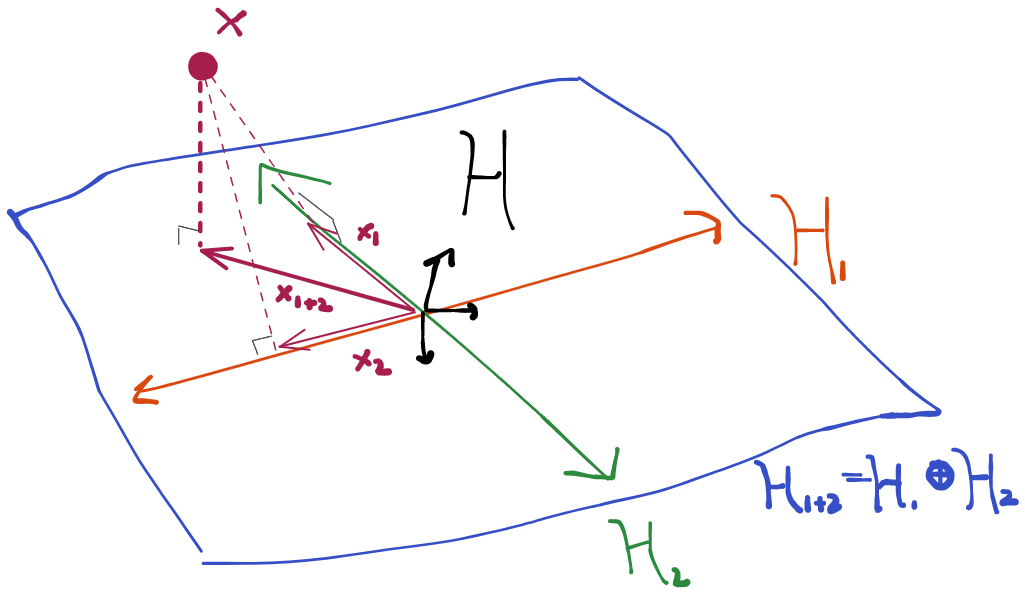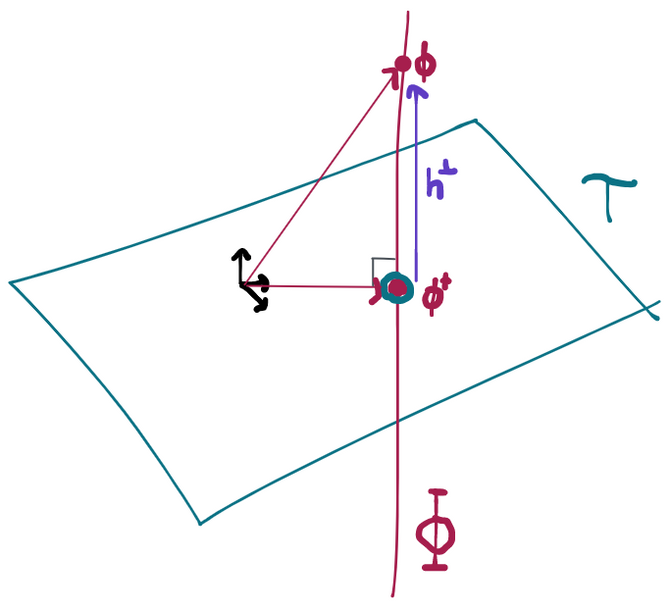And, in fact, this unique influence function $\phi^\dagger$﻿ in the tangent space is the one with the smallest variance. The proof of this is relatively simple: $\Phi$﻿ and $\mathcal T$﻿ are subsets of $\mathcal L_2^0$﻿. In this space, the norm is $||f||=E[f^2] = V[f]$﻿ since by definition $f$﻿ must have mean zero to be in $\mathcal L_2^0$﻿. Therefore looking for the smallest variance influence function is the same as looking for the influence function with the smallest norm in $\mathcal L_2^0$﻿, or, equivalently, the point in $\Phi$﻿ that's closest to the origin. We can write any point in $\phi \in \Phi$﻿ as the sum of the influence function that is in the tangent space plus some function that is orthogonal to the tangent set: $\phi = \phi^\dagger + h^\perp$﻿. But since those two components are at a right angle, there's no way for the length of the "vector" $\phi$﻿ to be less than the length of the "vector" $\phi^\dagger$﻿ because the Pythagorean theorem says that $||\phi||^2 = ||\phi^\dagger||^2 + ||h^\perp||^2$﻿.
🌈
Because $\phi^\dagger$﻿ has the smallest variance of any influence function, and therefore any RAL estimator that has it will make the most efficient possible use of the data, we call $\phi^\dagger$﻿ the efficient influence function (EIF; sometimes also referred to as efficient influence curve or EIC).
If we identify $\phi^\dagger$﻿ as the unique element in the tangent space for which the pathwise derivative in a particular direction can be represented as the inner product between that direction and this element (i.e. the Reisz representation), then it also makes sense to call $\phi^\dagger$﻿ the canonical gradient.
Why is it called the canonical gradient?
If we have a function $f:\mathbb R^p \rightarrow \mathbb R$﻿ that maps vectors to real numbers, the directional derivative at $\vec x$﻿ in the direction of a vector $\vec h$﻿ is defined as $\nabla_hf(\vec x) = \lim_{\epsilon \rightarrow 0} \frac{f(\vec x + \vec h) - f(\vec x) }{\epsilon}$﻿. However, it's well-known that we can also write this as the sum of the partial derivatives times the components of $\vec h$﻿: $\nabla_{\vec h} f(\vec x) = \frac{\partial f(\vec x)}{\partial x_1} h_1 + \dots + \frac{\partial f(\vec x)}{\partial x_p} h_p$﻿. In fact, the proof of this follows from noticing that the directional derivative is a linear operator and applying the Reisz representation theorem that we derived for finite dimensional $\mathbb R^p$﻿. Of course, this is the same as the inner product between the vector of partial derivatives, which we call the gradient $\nabla f(\vec x) = [\partial f(\vec x)/\partial x_1 \dots \partial f(\vec x)/\partial x_p]$﻿, and $h$﻿. Thus $\nabla_{\vec h} f = \vec h \cdot \nabla f = \langle \vec h, \nabla f \rangle_{\mathbb R^p}$﻿.
Now it should make sense why we refer to $\phi^\dagger$﻿ as a gradient. It's exactly the same as $\nabla f$﻿, except now we're in $\mathcal L_2^0$﻿ instead of $\mathbb R^p$﻿. Since we can write the directional derivative $\nabla_h \psi(P)$﻿ as an inner product $E[h, \phi^\dagger] = \langle h, \phi^\dagger \rangle_{\mathcal L_2}$﻿, the object $\phi^\dagger$﻿ is serving exactly the same role as the gradient $\nabla f$﻿ is above. To reiterate:
$\begin{array}{ccc} \nabla_{\vec h} f(\vec x) &=& \langle \vec h, \nabla f \rangle_{\mathbb R^p} \\ \underbrace{ \nabla_h \psi(P) }_ {\text{directional derivative}} &=& \underbrace{ \langle h, \phi^\dagger \rangle_{\mathcal L_2(P)} }_ {\text{inner product b/t direction and gradient}} \end{array}$
We call $\phi^\dagger$﻿ the canonical gradient because there are usually many functions in $\mathcal L_2$﻿ that satisfy the above (namely: every other influence function). However, $\phi^\dagger$﻿ is the only one that is in the tangent space, and is the only one that's guaranteed to exist according to the Reisz representation theorem. That's what makes it "canonical".
In the context we've described here it might make more intuitive sense to use the notation $\nabla \psi$﻿ to represent the canonical gradient. However, we use $\phi^\dagger$﻿ to make the connection to influence functions for RAL estimators. A gradient (depends on the parameter) and an influence function (depends on the estimator) are actually totally different things. It just so happens that for RAL estimators they happen to occupy exactly the same space.
If a model is saturated, these geometrical arguments imply that there is only one valid influence function (and it is therefore efficient). To see why, we'll assume that there are two different influence functions and then show that they are actually the same. If there are two IFs, we know that $E[(\phi_1 - \phi_2)h] = 0$﻿ for all $h$﻿ in the tangent set, which in the case of a saturated model is all of $\mathcal L_2^0$﻿. However, $\phi_1 - \phi_2$﻿ is itself a zero-mean, finite variance function (i.e. an element of the tangent set) so there is some score $h^* = \phi_1 - \phi_2$﻿. But by the orthogonality result, we must have that $E[(\phi_1 - \phi_2)h^*] = E[h^*h^*] = 0$﻿. Since nothing can be orthogonal to itself unless it is 0, we conclude that $\phi_1-\phi_2 = 0$﻿ and our two "different" influence functions are in fact the same one. This, in turn, means there exists only one RAL estimator (or, technically, one class of RAL estimators that are all asymptotically equivalent).